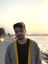Search
•Inan Evin

# #10 Lina Engine: Math Library - Part 1 Math & Vectors

Hello there guys, today I will be talking about the Math library for Lina Engine. The thing is, in order to perform basic operations like interpolations, smooth damping, clamping etc. as well as the complex operations like translation and rotation on objects, we need a solid math library written in our engine. We can not afford to create redundancies on the math calculations and cluttering up our code. We need solid structs that are able to do desired calculations for our operations. So for this, I have started writing a math class.

Initially, for operations like calculating sine and cosine as well as other trigonometric calculations, I will be using the default math library "cmath" in C++ for now. However, we need to put more into that, like distance, square root, determinant calculations etc. So I set up a basic math library, that are able to perform the initial calculations I need:

As you can see, I have not implemented much yet. I have a basic linear interpolation and a few utility methods that I will benefit in the future. The rest is already coming from the "cmath" library. However, what I really focused on is the Vector library. In order to do any kind of drawing on the screen, I would need vertices to operate with OpenGL. Most initially, I will need 3D and 2D space coordinate systems. For this, I have implemented a Vector2 and a Vector3 class. However, in order to perform drawing calculations properly in the future, I will need some core, fundamental functions. I can list them as:

Dot Product

Cross Product

Projection

Angle

Magnitude

Normalization

In order to properly implement drawing, we will need to normalize a lot of vectors in the future, as well as we will need angle calculations for camera and object rotations. For the dot product and cross product, well, we will need them everywhere. As an addition to these functions, I have implemented a bunch of overloaded methods for vector operations. I would like to have multiplication operations between copies of vectors, or on a single vector instance and in many more scenarios. I wanted to have a flexible syntax that works with both references of instances and copies of instance data. So, yes I did the most boring and painful part of C++ coding, overloading a bunch of operators. Below, you can find the implementation of the Vector3 class, if you would like to inspect. See you on the next post!